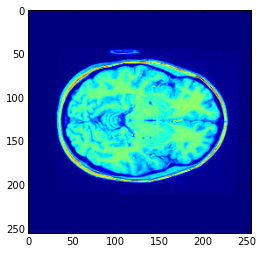What is an image?

We get an image from our example dataset : http://practical-neuroimaging.github.com/example_data.html

If we haven't got the zip archive already, we'll get it using Python

In :
import os # The Operating System module

In :
zip_fname = 'two_example_images.zip'

In :
if not os.path.isfile(zip_fname):
import urllib2 # library to get URLs
url = 'http://nipy.bic.berkeley.edu/practical_neuroimaging/' + zip_fname
# Get the data from the URL address
fileobj = urllib2.urlopen(url)
data = fileobj.read()
# Write it to disc as the zip file
local_fileobj = open(zip_fname, 'wb')
local_fileobj.write(data)
local_fileobj.close()


We now have the file downloaded locally. To check for the file, we can use the glob module. This allows us to do wildcard matching to filenames. For example, to look for files in the current directory ending with .zip:

In :
import glob
glob.glob('*.zip')

Out:
['two_example_images.zip']

What does this file have inside? We can look at it using the Python zipfile module.

In :
import zipfile
z_fobj = zipfile.ZipFile(zip_fname, 'r')
z_fobj.namelist()

Out:
['ds107_sub001_highres.nii.gz', 'ds107_sub001_run01.nii.gz']

Don't forget to tab complete on - say - z_fobj above, to see what you can do with it.

We extract the files:

In :
z_fobj.extractall()
z_fobj.close()
glob.glob('*.nii.gz')

Out:
['ds107_sub001_highres.nii.gz', 'ds107_sub001_run01.nii.gz']

The images have gz extensions. Looks like they are gzipped. What could be better than opening the file with the gzip module?

In :
import gzip
gzfobj = gzip.open('ds107_sub001_highres.nii.gz', 'rb')


What type of thing is gzfobj? Try typing Ctrl-m b to insert a cell below, then type gzfobj. (remember the dot) and then tab.

Let's read in all the data to take a look

In :
img_data = gzfobj.read()
gzfobj.close()


What do we have now?

In :
type(img_data)

Out:
str
In :
len(img_data)

Out:
25166176

What is in here? I do a Google search for "Nifti header". The second hit takes me to this page: http://nifti.nimh.nih.gov/nifti-1/documentation/nifti1fields.

It reveals that that first bytes should be an integer value 348 and that it has a length of 4:

int sizeof_hdr; /*!< MUST be 348 */ /* int sizeof_hdr; */ /* 0 */

Let's look at the numerical values of the first 4 bytes:

In :
for i in range(4):
print ord(img_data[i])

92
1
0
0


This is 348 as a four byte integer. Quick quiz: why? Clue follows:

In :
348 - 92

Out:
256

OK, so we want to put this binary form of the integer in memory, into Python as an integer object. How to do that?

In :
import struct


To see what is in struct, try Ctrl-m b again, and struct? to see the help. Then check struct.pack? and struct.unpack?. Among other things, we find that <i is the code for a signed 4-byte 32-bit integer, with little-endian byte ordering.

In :
struct.unpack('<i', img_data[:4])

Out:
(348,)

Nice. Now, what else do we need to know? The shape of the data? We get this from this field of the NIfTI header:

short dim; /*!< Data array dimensions.*/ /* short dim; */ /* 40 */

The help for struct tells us we need the code <8h for 8 16-bit little-endian integers.

In :
struct.unpack('<8h', img_data[40:56])

Out:
(3, 256, 256, 192, 1, 1, 1, 1)

OK - looks like it's 256 by 256 by 192. What's the data type? We want this field:

short datatype; /*!< Defines data type! */ /* short datatype; */ /* 70 */

In :
struct.unpack('<h', img_data[70:72])

Out:
(4,)

Looking at : http://nifti.nimh.nih.gov/nifti-1/documentation/nifti1fields/nifti1fields_pages/datatype.html - this seems to mean 16 bit signed integer. Where does the image data start in this file?

float vox_offset; /*!< Offset into .nii file */ /* float vox_offset; */ /* 108 */

The code we need is for a 4-byte floating point value - <f

In :
struct.unpack('<f', img_data[108:112])

Out:
(352.0,)

That's what we were expecting (well - it was what I was expecting!).

So, we are expecting 256 256 192 2-byte integers, to follow the 348 bytes of the header, and the 4 extra bytes before the data starts. So how long should the entire image file be?

Now we can could read the image data, veerrryyy slowly, like this:

In :
fmt_str = '<%dh' % (256 * 256 * 192)
img_values = struct.unpack(fmt_str, img_data[352:])

In :
len(img_values)

Out:
12582912
In :
type(img_values)

Out:
tuple

We can get the mean of all the values in the image like this:

In :
sum(img_values) / float(len(img_values))

Out:
32.01169069608053

It's time to introduce numpy. Numpy is the array manipulation library for Python

In :
import numpy as np


Numpy allows you to look at binary data as arrays. For example, here's a much quicker trick to get the image data into an array:

In :
img_array = np.ndarray(shape=(256, 256, 192), dtype='<i2', order='F', buffer=img_data[352:])


Please don't worry about the details of this command, but what we get back is - an array:

In :
img_array.shape

Out:
(256, 256, 192)
In :
img_array.mean()

Out:
32.011690696080528

As we'll see soon, we can take slices out of this array to show image slices. First we enable the plotting. Don't worry about the details, we'll go over this again:

In :
%pylab inline

Welcome to pylab, a matplotlib-based Python environment [backend: module://IPython.zmq.pylab.backend_inline].
For more information, type 'help(pylab)'.

In :
z_slice = img_array[:, :, 96]
plt.imshow(z_slice)

Out:
<matplotlib.image.AxesImage at 0x105c2a7d0>